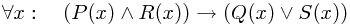This is a little note on how there's an enormous gap between what the logical symbol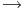for (material) implication (or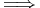, though there's the possibility of distinguishing these...) means, and what humans mean when they say something implies something. (This came up here.)

In a logical setting,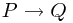is exactly the same as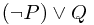. This might look a little surprising the first time you see it, but the reasoning is as follows: "P implies Q" says that "it's impossible to have P without having Q"; that is,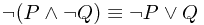. Alternatively, reading it directly, "either the condition P doesn't hold, or Q does".

You can already start to see why this is a little odd. For instance, suppose Q was just "true". Thenis also true, whatever we choose P to be. For instance,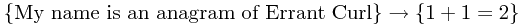Or if the condition P is false, we reach a similar conclusion, as in the true statement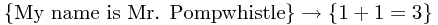In general, this sort of implication doesn't mean anything like what you do when you say 'implies'. Most people are thinking of some causal or at least relevant implication; intuitively, you intend firstly that the condition can at least in principle be true, and preferably that the conclusion can similarly be false, and that somehow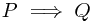means that P being true tells you something which needn't have been true; in particular, that Q follows non-trivially from P.

Where things start getting really weird, though, is when we start having implications as part of a larger logical expression. Let's plod through a calculation and see where it gets us, then try to understand why this happens.

Consider the expression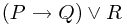which looks simple enough. Let's expand this out: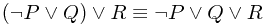Suddenly, the fact that all of these terms are being treated on equal ground looks a little strange. We could regroup these 'or' terms in lots of different ways. For example,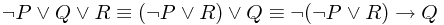or to wrap it all up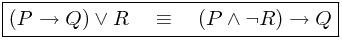Is this weird? Let's make up something such that the right-hand side is true. For instance, P is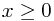, R is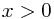and Q is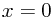. Then indeed, "P and not Q" implies Q.

We've shown that this is equivalent to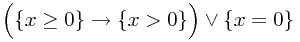so this must be true.

... oh. It doesn't look like either statement is necessarily true, given an arbitrary x, but apparently one of them is! But we can choose x to be non-zero, which violates the second term; and if we choose x to be zero, we violate the first term!

Of course, we haven't broken logic. Instead, we've got confused about quantifiers, most likely because we were lazy and didn't write them. What we've really shown is thatNow notice that given some x, it is true that either it is 0, or - should it be at least zero - it is strictly bigger than zero! Good, we've made sense of it.

That wasn't so hard to resolve. However, things can look much odder than this. Here's an example:

Consider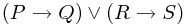- one of two implications is true.

We can expand this out as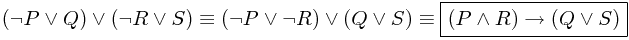Note that this is equivalent to our original statement; it's true if and only if the original one holds. Okay, fine.

Let's make something up. Suppose our dictionary is the following:

• P: It's raining
• R: I'm outside
• Q: I have an umbrella
• S: I'm drenched

So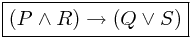just says

If (it's raining and I'm outside) then (either I have an umbrella or I'm drenched).

Let's drag back the thing it's supposed to be equivalent to:. What does this say?

It is the case that either (if it's raining then I have an umbrella) or (if I'm outside I'm drenched).

...?! Neither of these implications looks remotely sensible on its own, but we've seen that one of them is true, if our original (very reasonable) assessment was true!

We'd say "if it's raining, then I can simply choose not to have an umbrella"; or "if I'm outside, then maybe it's not raining, or maybe I brought an umbrella"! These seem perfectly reasonable objections, but logically we're being told that one of the implications is true.

The fallacy arises when we carelessly think, "I can take the implication out, and view it on its own, and then consider all possible cases that could be relevant to see if it's true". This is emphatically not the case! Even though when any normal person says "(A implies B) or (C implies D)", they mean that in general a particular one of those two implications holds, logically, that's not what our expression means.

What it means is that, on any given day, given what I'm doing and what state I'm in, one of these implications holds. This might be completely uninformative. For example, suppose that, whatever else is true, I have an umbrella. Then the mysterious "(if it's raining then I have an umbrella) or (if I'm outside I'm drenched)" is actually trivially satisfied, since it is true that I have an umbrella, which makes the first bracket true!

Or perhaps just imagine I'm inside. Then, once more, the expression becomes magically true, since violating a condition makes an implication trivially true.

By contrast, if you make one of the implications false, then the other one becomes sensible once more: imagine it's raining but you don't have an umbrella. This makesfalse, as a description of the world as it is. But now the only possibility is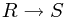:

[Given that it's raining but you don't have an umbrella] if you're outside, then you are drenched.

So regardless of what sort of thing you do, the statement turns out to make logical sense. However, it is crucial it is viewed as a whole. You can make the other implication true just by choosing a different description! Flipping the above, for example,

[Given that you're outside, but not drenched] if it's raining, then you have an umbrella.

Once more, the secret is missing quantifiers! All the propositions P, Q, R, S are functions of the world's state x, and the quantifier x appears outside everything as a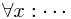- it's crucial to realize you can't push this inside the subexpressions! It's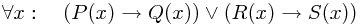NOT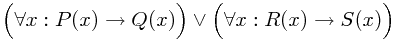which is equivalent to